International
Tables for
Crystallography
Volume D
Physical properties of crystals
Edited by A. Authier

International Tables for Crystallography (2013). Vol. D, ch. 1.11, pp. 276-277

## Section 1.11.6.2. Tensor atomic factors (non-magnetic case)

V. E. Dmitrienko,a* A. Kirfelb and E. N. Ovchinnikovac

aA. V. Shubnikov Institute of Crystallography, Leninsky pr. 59, Moscow 119333, Russia,bSteinmann Institut der Universität Bonn, Poppelsdorfer Schloss, Bonn, D-53115, Germany, and cFaculty of Physics, M. V. Lomonosov Moscow State University, Leninskie Gory, Moscow 119991, Russia
Correspondence e-mail:  dmitrien@crys.ras.ru

#### 1.11.6.2. Tensor atomic factors (non-magnetic case)

| top | pdf |

In time-reversal invariant systems, equation (1.11.6.3)can be rewritten aswhere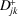corresponds to the symmetric part of the dipole–dipole contribution,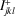andmean the symmetric and antisymmetric parts of the third-rank tensor describing the dipole–quadrupole term, and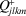denotes a symmetric quadrupole–quadrupole contribution. From the physical point of view, it is useful to separate the dipole–quadrupole term intoand, because in conventional optics, where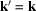, onlyis relevant.

The tensors contributing to the atomic factor in (1.11.6.16),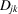,,,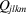, are of different ranks and must obey the site symmetry of the atomic position. Generally, the tensors can be different, even for crystallographically equivalent positions, but all tensors of the same rank can be related to one of them, because all are connected through the symmetry operations of the crystal space group. In contrast, the scattering amplitude tensor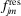does not necessarily comply with the point symmetry of the atomic position, because this symmetry is usually violated considering the arbitrary directions of the radiation wavevectorsand.

Equation (1.11.6.16)is also frequently considered as a phenomenological expression of the tensor atomic factor where each tensor possesses internal symmetry (with respect to index permutations) and external symmetry (with respect to the atomic environment of the resonant atom). For instance, the tensoris symmetric, the rank-3 tensor has a symmetric and a antisymmetric part, and the rank-4 tensor is symmetric with respect to the permutation of each pair of indices. The external symmetry ofcoincides with the symmetry of the dielectric susceptibility tensor (Chapter 1.6). Correspondingly, the third-rank tensorsandare similar to the gyration susceptibility and electro-optic tensors (Chapter 1.6), andhas the same tensor form as that for elastic constants (Chapter 1.3). The symmetry restrictions on these tensors (determining the number of independent elements and relationships between tensor elements) are very important and widely used in practical work on resonant X-ray scattering. Since they can be found in Chapters 1.3and 1.6or in textbooks (Sirotin & Shaskolskaya, 1982; Nye, 1985), we do not discuss all possible symmetry cases in the following, but consider in the next section one specific example for X-ray scattering when the symmetries of the tensors given by expression (1.11.6.3)do not coincide with the most general external symmetry that is dictated by the atomic environment.

### References

Nye, J. F. (1985). Physical Properties of Crystals: Their Representation by Tensors and Matrices. Oxford University Press.Google Scholar
Sirotin, Y. & Shaskolskaya, M. P. (1982). Fundamentals of Crystal Physics. Moscow: Mir.Google Scholar